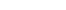Loading... Please wait...

# BUS 308 Week 1 Assignment Problem Set Week One (New 2015)

Price:
\$25.00

Click the button below to add the BUS 308 Week 1 Assignment Problem Set Week One (New 2015) to your wish list.

SHARE

## Product Description

BUS 308 Week 1 Assignment Problem Set Week One

Assignment: Problem Set Week one

All statistical calculations will use the Employee Salary Data set included in the online course.

1. For assistance with these calculations, see the Recommended Resources for Week One. Measurement issues. Data, even numerically code variables, can be one of 4 levels – nominal, ordinal, interval, or ratio. It is important to identify which level a variable is, as this impacts the kind of analysis we can do with the data. For example, descriptive statistics such as means can only be done on interval or ratio level data. Please list, under each label, the variables in our data set that belong in each group.
2. The first step in analyzing data sets is to find some summary descriptive statistics for key variables. For salary, compa, age, Performance Rating, and Service; find the mean and standard deviation for 3 groups: overall sample, Females, and Males. You can use either the Data Analysis Descriptive Statistics tool or the Fx =average and =stdev functions. Note: Place data to the right, if you use Descriptive statistics, place that to the right as well.
3. What is the probability for a:
1. Randomly selected person being a male in grade E?
2. Randomly selected male being in grade E?
3. Why are the results different?
4. For each group (overall, females, and males) find:
1. The value that cuts off the top 1/3 salary in each group.
2. The z score for each value.
3. The normal curve probability of exceeding this score.
4. What is the empirical probability of being at or exceeding this salary value?
1. The score that cuts off the top 1/3 compa in each group.
2. The z score for each value.
3. The normal curve probability of exceeding this score.
4. What is the empirical probability of being at or exceeding this salary value?
5. How do you interpret the relationship between the data sets? What do they mean about our equal pay for
equal work question?
5. a. What conclusions can you make about the issue of male and male pay equality? Are all of the results consistent?
b. c. d. e. f.

What is the difference between the salary and compa measures of pay? Conclusions from looking at salary results:
Conclusions from looking at compa results:
Do both salary measures show the same results?
Can we make any conclusions about equal pay for equal work yet?
assistance with these calculations, reference the Recommended Resources for Week One.

## Product Reviews

This product hasn't received any reviews yet. Be the first to review this product!

• \$20.00
• \$10.00
• \$25.00
• \$5.00
• \$10.00

• \$20.00
• \$10.00
• \$25.00
• \$5.00
• \$10.00

## Related Products

• \$15.99• \$20.00• \$20.00• \$20.00• \$20.00